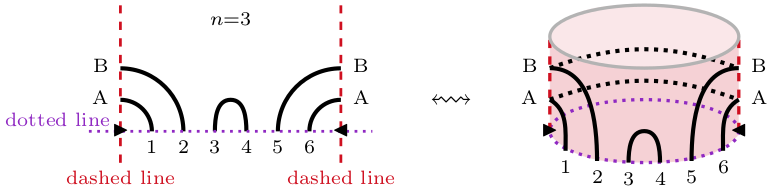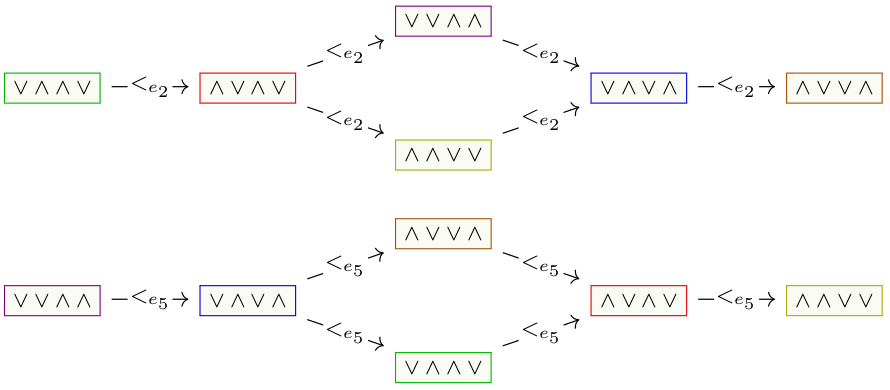## Data

• Title: Relative cellular algebras
• Authors: Michael Ehrig and Daniel Tubbenhauer
• Status: Transform. Groups 26 (2021), no. 1, 229-277. Last update: Thu, 12 Sep 2019 08:38:59 UTC
• ArXiv version = 0.99 published version

## Abstract

In this paper we generalize cellular algebras by allowing different partial orderings relative to fixed idempotents. For these relative cellular algebras we classify and construct simple modules, and we obtain other characterizations in analogy to cellular algebras.
We also give several examples of algebras that are relative cellular, but not cellular. Most prominently, the restricted enveloping algebra and the small quantum group for $$\mathfrak{sl}_{2}$$, and an annular version of arc algebras.

## A few extra words

In pioneering work Graham-Lehrer introduced the notion of a cellular algebra, i.e. an algebra equipped with a so-called cell datum. For example, of key importance for this paper, the cell datum comes with a set $$\mathsf{X}$$ and a partial order $$<$$ on it.
The cell datum provides a method to systematically reduce hard questions about the representation theory of such algebras to problems in linear algebra. Hereby the partial order $$<$$ on $$\mathsf{X}$$ plays an important role since it yields an “upper triangular way” to construct certain modules, called \textit{cell modules}, which have a crucial role in the theory.
In well-behaved cases the linear algebra problems can be solved giving e.g. a parametrization of the isomorphism classes of simple modules via a subset of $$\mathsf{X}$$, and a construction of a representative for each class.
In this paper we (strictly) generalize the notion of a cellular algebra to what we call a relative cellular algebra, i.e. an algebra equipped with a relative cell datum. For example, the relative cell datum comes with a set $$\mathsf{X}$$, but now with several partial orders $$<_{\boldsymbol{\varepsilon}}$$ on it, one for each idempotent $$\boldsymbol{\varepsilon}$$ from a preselected set of idempotents. Taking only one idempotent $$\boldsymbol{\varepsilon}=1$$, namely the unit, and only one partial order $$<_{1}=<$$, we recover the setting of Graham-Lehrer.
Surprisingly, most of the theory of cellular algebras still works in this relative setup. However, with fairly different proofs, carefully incorporating the various partial orders. The purpose of our paper is to explain this in detail.
One of our main examples of an algebra which is relative cellular, but not cellular in the usual sense is an annular version of arc algebras with the following picture to be kept in mind:For this algebra there are various partial orderings $$<_{e_i}$$ (on the same set) attached to certain idempotents $$e_i$$. Here an example of how these partial orderings can vary.